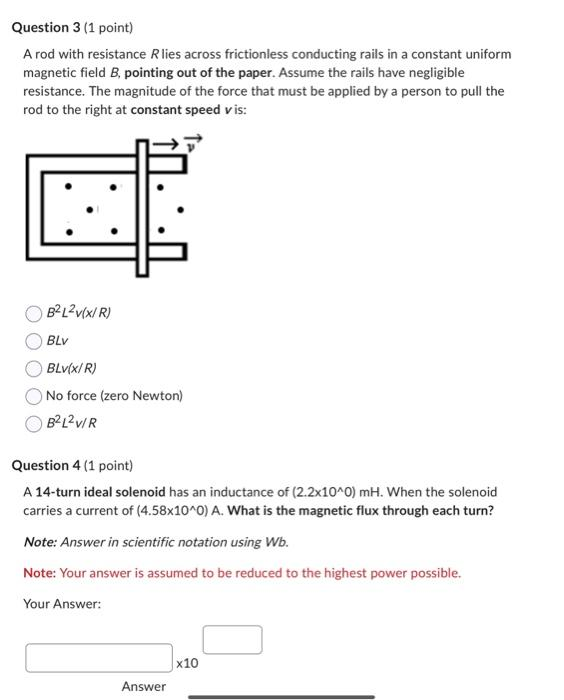# (Solved): A rod with resistance R lies across frictionless conducting rails in a constant uniform magnetic fi ...A rod with resistance lies across frictionless conducting rails in a constant uniform magnetic field , pointing out of the paper. Assume the rails have negligible resistance. The magnitude of the force that must be applied by a person to pull the rod to the right at constant speed is: No force (zero Newton) Question 4 (1 point) A 14-turn ideal solenoid has an inductance of . When the solenoid carries a current of . What is the magnetic flux through each turn? Note: Answer in scientific notation using . Note: Your answer is assumed to be reduced to the highest power possible. Your Answer: Answer

We have an Answer from Expert